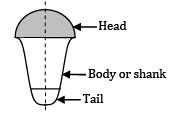Courses

# Test: Design Of Joints (Level - 1)

## 15 Questions MCQ Test Machine Design | Test: Design Of Joints (Level - 1)

Description
This mock test of Test: Design Of Joints (Level - 1) for Mechanical Engineering helps you for every Mechanical Engineering entrance exam. This contains 15 Multiple Choice Questions for Mechanical Engineering Test: Design Of Joints (Level - 1) (mcq) to study with solutions a complete question bank. The solved questions answers in this Test: Design Of Joints (Level - 1) quiz give you a good mix of easy questions and tough questions. Mechanical Engineering students definitely take this Test: Design Of Joints (Level - 1) exercise for a better result in the exam. You can find other Test: Design Of Joints (Level - 1) extra questions, long questions & short questions for Mechanical Engineering on EduRev as well by searching above.
QUESTION: 1

Solution:
QUESTION: 2

Solution:
QUESTION: 3

### A butt welded joint, subjected to tensile force P is shown in the given figure, l length of the weld (in mm), h throat of the butt weld (in mm) and H is the total height of weld including reinforcement. The average tensile stress , in the weld is given by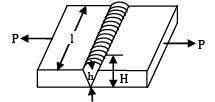Solution:

Note – Height of reinforcement is not considered in the stress calculations

QUESTION: 4

The thickness of a boiler plate is 16 mm, the diameter of rivet used in the boiler joint is

Solution:

Thickness of boiler plate,

t = 16mm

Using Unwin’s equation,

Diameter of rivet (d) = 6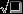= 6= 24

QUESTION: 5

The distance between the centers of the rivets in adjacent rows of zigzag riveted joint is known as __________.

Solution:
QUESTION: 6

Rivets are generally specified by

Solution:

Rivets are generally specified by nominal diameter.

QUESTION: 7

A double strap butt joint is always in

(A) single shear

(B) double shear

(C) either single or double shear

(D) None of the above

Solution:
QUESTION: 8

The rivet head for general purpose shown in the figure below is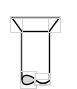Solution: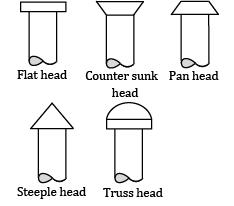QUESTION: 9

Rivets are made of

Solution:
QUESTION: 10

In a single V-butt welds, the angle between edges is kept about

Solution:

In a single V-butt weld, the angle between the edges is kept about.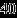to 60о

QUESTION: 11

The shear strength, tensile strength and compressive strength of a rivet joint are 100 N, 120 N and 150 N respectively. If strength of the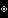unriveted plate is 200 N, the efficiency of rivet joint is

Solution:

Shear strength, PS = 100 N

Tensile strength, Pt = 120 N

Compressive strength, Pc = 190 N

Strength of unriveted plate, P = 200 N

Efficiency of riveted joint is defined as ratio of the strength of riveted joint to the strength of unriveted solid plate.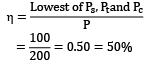QUESTION: 12

The shearing strength of a rivet in 50 N. If the diameter of the rivet is doubled, then its shearing strength will be

(A)100 N

Solution:

Shearing strength of rivet by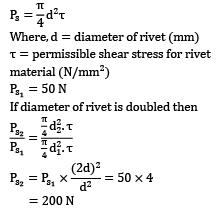Where, d = diameter of rivet (mm)

τ = permissible shear stress for rivet material (N/mm2)

Ps1 = 50 N

If diameter of rivet is doubled then= 200 N

QUESTION: 13

If the tearing efficiency of a riveted joint is 60%, then the ratio of rivet hole diameter to the pitch of rivets is __________.

Solution: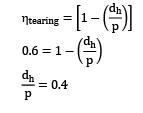QUESTION: 14

A riveted joint may fail due to

A. Shearing of the rivet

B. Shearing off the plate at an angle

C. Crushing of the rivet

Solution:
QUESTION: 15

Aircraft body is usually fabricated by

Solution:

Rivet is a short cylindrical bar with a head with it. It consists of three parts

(A) Head

(B) Body/shank

(C) Tail